Question

Need help with this moment of inertia problem. Please show all work For the shape below,...

Need help with this moment of inertia problem. Please show all work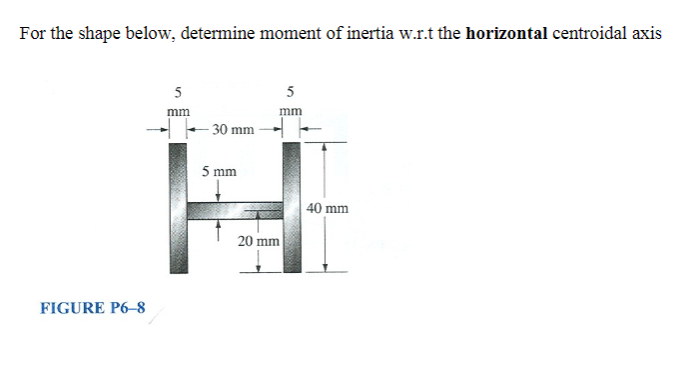For the shape below, determine moment of inertia w.r.t the horizontal centroidal axis 30 mm 5 mm 40 mm 20 mm FIGURE P6-8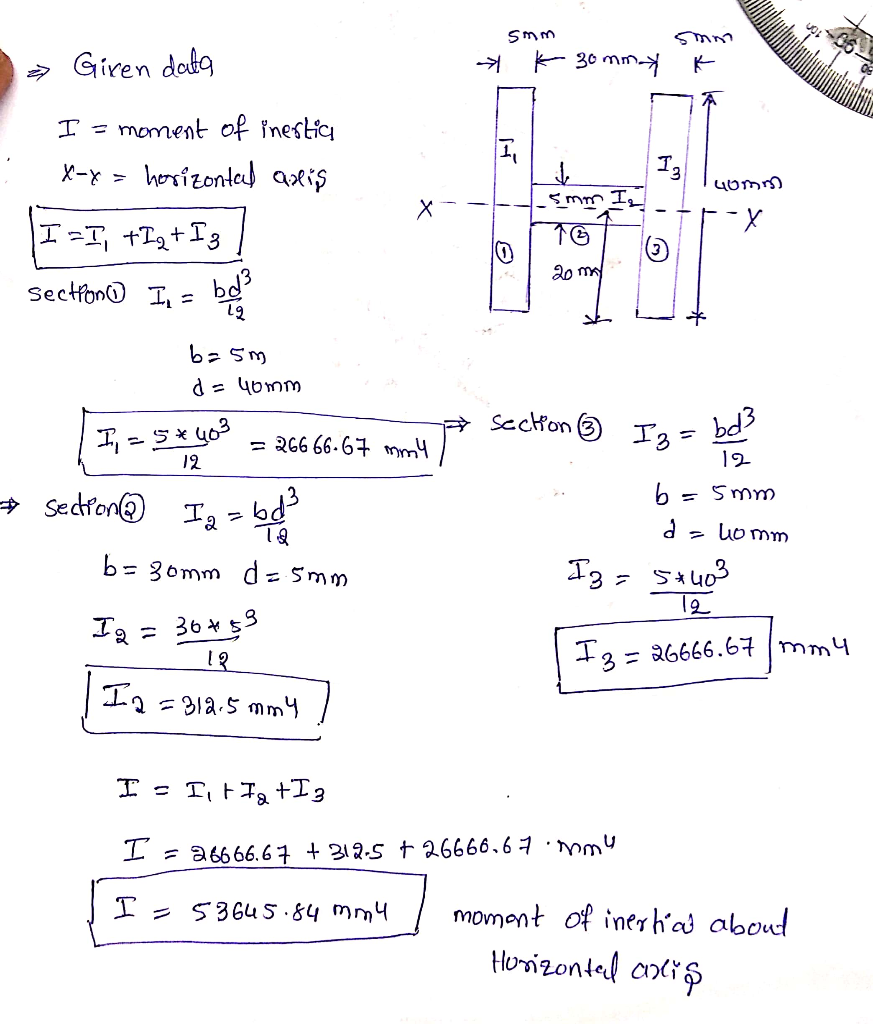plz like

Earn Coins

Coins can be redeemed for fabulous gifts.

Similar Homework Help Questions
• please show all your work thank you! Problem 2 (25%) 14 in A beam cross-section is...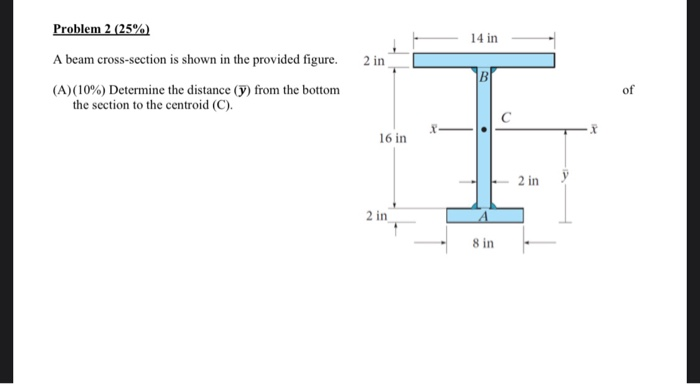please show all your work thank you! Problem 2 (25%) 14 in A beam cross-section is shown in the provided figure. 2 in (A)(10%) Determine the distance (y) from the bottom the section to the centroid (C). 16 in 8 in Problem 2 (25% - 14 in- A beam cross-section is shown in the provided figure. 2 in (B) (15%) Determine the moment of inertia of the shape about the X-axis (i.e. the horizontal centroidal axis) 16 in - 2...

• Please show all work. Correct answer should be H. Will rate! 20) The y-coordinate of the centroid of the shaded area is 5.167 in. above the bottom edge of the section. The area moment of inertia abou...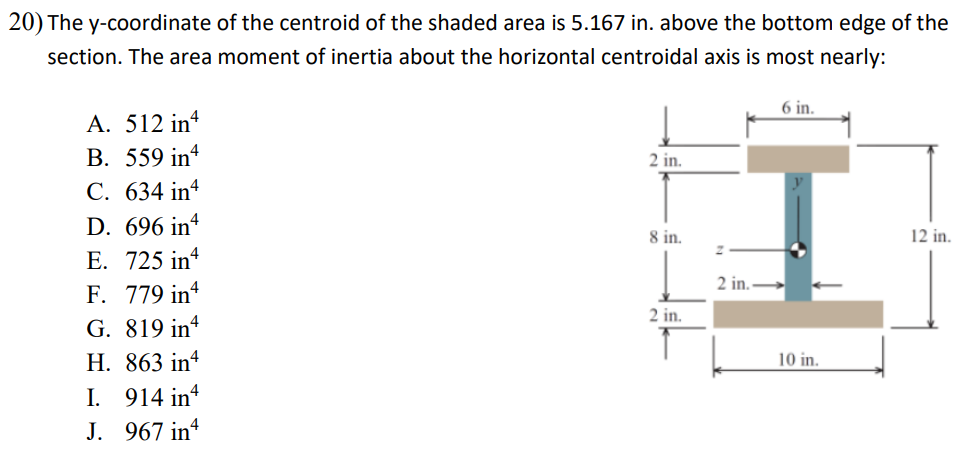Please show all work. Correct answer should be H. Will rate! 20) The y-coordinate of the centroid of the shaded area is 5.167 in. above the bottom edge of the section. The area moment of inertia about the horizontal centroidal axis is most nearly: 6 in 4 A. 512 in B. 559 in 2 in. C. 634 in D. 696 in E. 725 in 8 in. in 2 in. F. 779 in G. 819 in 2 in H. 863 in...

• just need #6 (5) 12 mm 12 mm Determine the moment of inertia and the radius...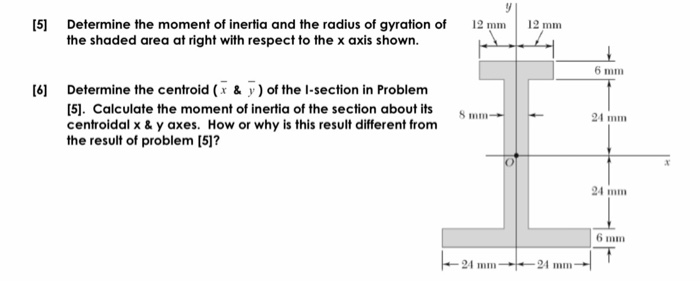just need #6 (5) 12 mm 12 mm Determine the moment of inertia and the radius of gyration of the shaded area at right with respect to the x axis shown. 6 mm  Determine the centroid (x & y) of the I-section in Problem (5). Calculate the moment of inertia of the section about its centroidal x & y axes. How or why is this result different from the result of problem (5]? S mm- 21 mm 6 mm...

• Problem 1 Determine the moment of inertia of the T section shown below with respect to...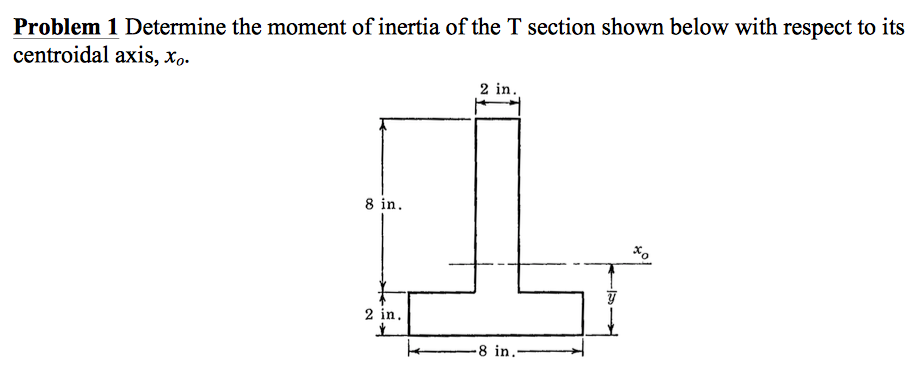Problem 1 Determine the moment of inertia of the T section shown below with respect to its centroidal axis, xo 2 in 8 in 2 in -8 in

• statics help( please show work so i can understand) Determine the moment of inertia, Ix (not...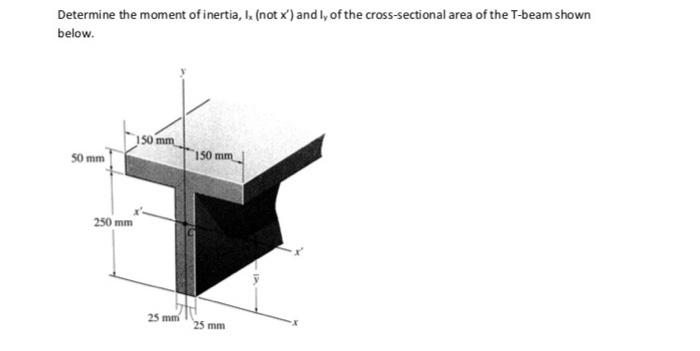statics help( please show work so i can understand) Determine the moment of inertia, Ix (not x) and ly of the cross-sectional area of the T-beam shown below. 150 mm SO mm 150 mm 250 mm 25 mm 25 mm

• Learning Goal: To be able to calculate the moment of inertia of composite areas An object's...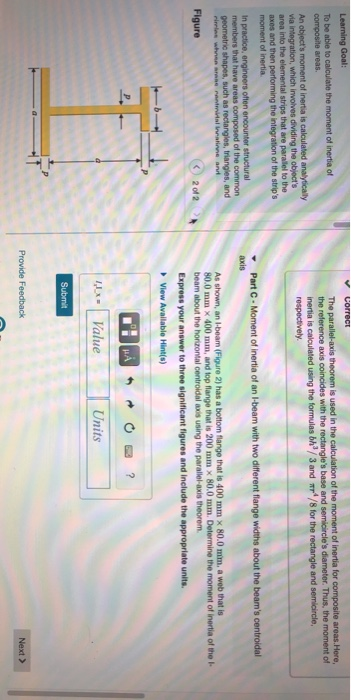Learning Goal: To be able to calculate the moment of inertia of composite areas An object's moment of inertia is calculated analytically via Integration, which involves dividing the object's aren into the elemental strips that are parallel to the axes and then performing the integration of the strip's moment of inertia correct The parallel-axis theorem is used in the calculation of the moment of inertia for composite areas. Here, the reference axis coincides with the rectangle's base and semicircle's diameter....

• 5. Determine the Moment of Inertia Ix and Ty of the composite cross section about the...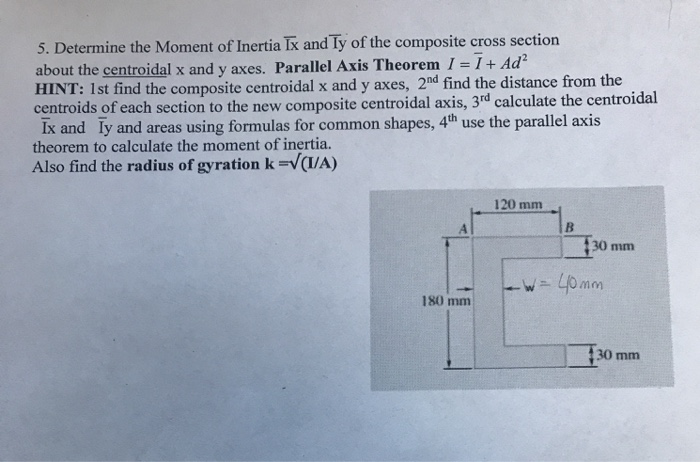5. Determine the Moment of Inertia Ix and Ty of the composite cross section about the centroidal x and y axes. Parallel Axis Theorem I = 7+ Ad HINT: 1st find the composite centroidal x and y axes, 2nd find the distance from the centroids of each section to the new composite centroidal axis, 3rd calculate the centroidal Ix and ly and areas using formulas for common shapes, 4th use the parallel axis theorem to calculate the moment of inertia....

• Moments of Inertia for Composite Areas Part A Moment of Inertia of a Composite Beam about...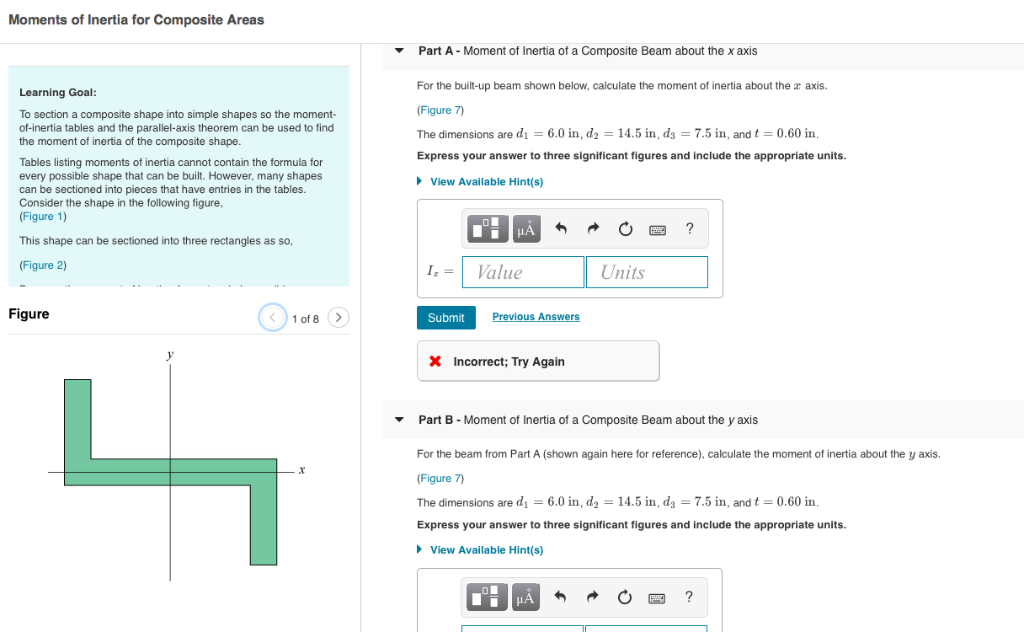Moments of Inertia for Composite Areas Part A Moment of Inertia of a Composite Beam about the x axis For the built-up beam shown below, calculate the moment of inertia about the r axis. (Figure 7) The dimensions are d1 = 6.0 in, d2 = 14.5 in, ds = 7.5 in, and t = 0.60 in. Express your answer to three significant figures and include the appropriate units. Learning Goal To section a composite shape into simple shapes so the...

• (10 points) Determine the moment of inertia of the composite beam about the centroidal x and...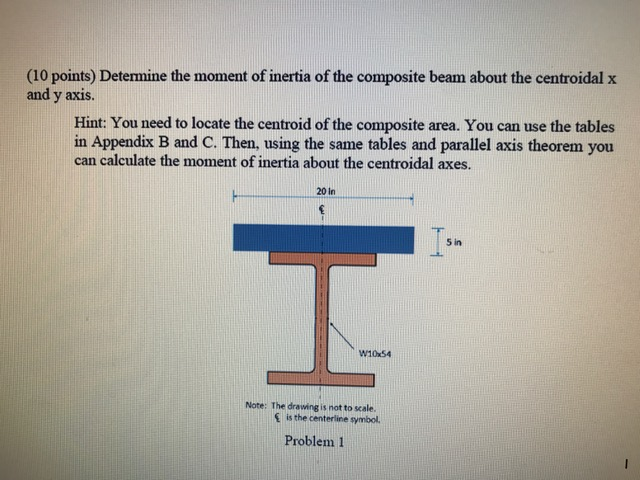(10 points) Determine the moment of inertia of the composite beam about the centroidal x and y axis. Hint: You need to locate the centroid of the composite area. You can use the tables in Appendix B and C. Then, using the same tables and parallel axis theorem you can calculate the moment of inertia about the centroidal axes. 20 in Ism 5 in W10x54 Note: The drawing is not to scale. is the centerline symbol Problem 1

• I need help on this Static problem Thank you! Using the parallel-axis theorem, determine the product...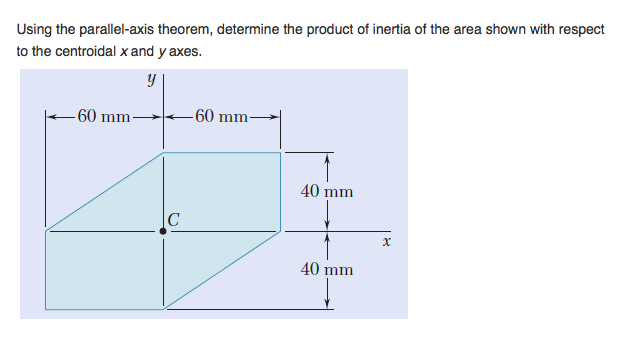I need help on this Static problem Thank you! Using the parallel-axis theorem, determine the product of inertia of the area shown with respect to the centroidal x and y axes. 60 mm-60 mm 40 mm 40 mm